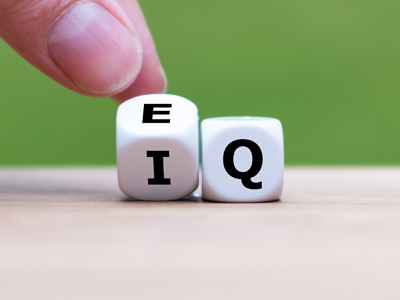EQ is for Education Quizzes. IQ is for intelligence quotient.

# Following Instructions 4

Congratulations – you have made it to the final test in our 11+ Verbal Reasoning series of quizzes on Following Instructions With Figures. If you have played the previous three, then you will know what is meant by terms such as product, quotient or sum. If not, then please try those ones first. The more practise you get with these kinds of question, the more prepared you will be for your exams.

These questions do require a little bit of maths, despite being in this section. But don’t worry – none of them are too testing. So long as you understand the questions and have a reasonably strong ability in maths, you’ll be fine.

I can’t stress enough how important it is that you read each question carefully before you choose your answer. This is a test of concentration as much a anything else. Good luck!

Example:
Multiply the first figure by the sum of the last two:
8, 5, 4

The sum of the last two digits is 9 (5 + 4 = 9). The answer is 9 x 8 = 72
1.
Add the last figure to the product of the first two:
11, 2, 43
65
56
74
47
The product of the first two figures is 22 (11 x 2 = 22). The answer is 22 + 43 = 65
2.
Subtract the first figure from the quotient of the last two (the last figure is the dividend and the middle one is the divisor):
16, 3, 60
-4
4
17
-17
The quotient of the last two figures is 20, if 60 is the dividend and 3 the divisor (60 ÷ 3 = 20). The answer is 20 - 16 = 4
3.
Multiply the first figure by the sum of the last two:
21, 3, 7
168
441
210
70
The sum of the last two digits is 10 (3 + 7 = 10). The answer is 10 x 21 = 210
4.
Subtract the first figure from the product of the last two:
100, 7, 19
-74
74
44
33
The product of the last two figures is 133 (7 x 19 = 133). The answer is 133 - 100 = 33
5.
Subtract the first figure from the second, then multiply your answer by the third:
26, 45, 3
-57
57
58
59
The second figure minus the first is 19 (45 - 26 = 19). The answer is 19 x 3 = 57
6.
Add the first figure to the product of the last two:
48, 3, 15
66
103
96
93
The product of the last two digits is 45 (3 x 15 = 45). The answer is 45 + 48 = 93
7.
Multiply the last figure by the sum of the first two:
8, 17, 8
272
144
200
288
The sum of the first two figures is 25 (8 + 17 = 25). The answer is 25 x 8 = 200
8.
Add the first figure to the quotient of the last two (the middle figure is the dividend and the last is the divisor):
78, 56, 4
87
90
91
92
The quotient of the last two figures is 14, if 56 is the dividend and 4 the divisor (56 ÷ 4 = 14). The answer is 78 + 14 = 92
9.
Subtract the third figure from the quotient of the first two (the first figure is the dividend and the second is the divisor):
144, 6, 13
15
11
14
12
The quotient of the first two figures is 24, if 144 is the dividend and 6 the divisor (144 ÷ 6 = 24). The answer is 24 - 13 = 11
10.
Multiply the second figure by the sum of the first and last:
9, 8, 6
120
432
126
102
The sum of the first and last figures is 15 (9 + 6 = 15). The answer is 15 x 8 = 120
Author:  Stephen O'Hara and Ian Miles